# Stable distribution

(diff) ← Older revision | Latest revision (diff) | Newer revision → (diff)

A probability distribution with the property that for any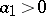,,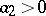,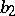, the relation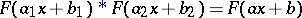(1)

holds, where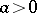andis a certain constant,is the distribution function of the stable distribution andis the convolution operator for two distribution functions.

The characteristic function of a stable distribution is of the form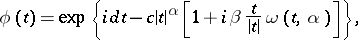(2)

where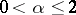,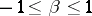,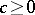,is any real number, and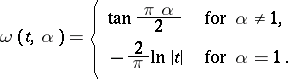The numberis called the exponent of the stable distribution. A stable distribution with exponentis a normal distribution, an example of a stable distribution with exponent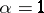is the Cauchy distribution, a stable distribution which is a degenerate distribution on the line. A stable distribution is an infinitely-divisible distribution; for stable distributions with exponent,, one has the Lévy canonical representation with characteristic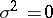,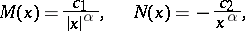whereis any real number.

A stable distribution, excluding the degenerate case, possesses a density. This density is infinitely differentiable, unimodal and different from zero either on the whole line or on a half-line. For a stable distribution with exponent,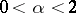, one has the relationsfor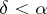, whereis the density of the stable distribution. An explicit form of the density of a stable distribution is known only in a few cases. One of the basic problems in the theory of stable distributions is the description of their domains of attraction (cf. Attraction domain of a stable distribution).

In the set of stable distributions one singles out the set of strictly-stable distributions, for which equation (1) holds with. The characteristic function of a strictly-stable distribution with exponent(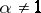) is given by formula (2) with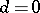. Fora strictly-stable distribution can only be a Cauchy distribution. Spectrally-positive (negative) stable distributions are characterized by the fact that in their Lévy canonical representation(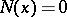). The Laplace transform of a spectrally-positive stable distribution exists if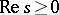:whereis the density of the spectrally-positive stable distribution with exponent,,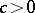,is a real number, and those branches of the many-valued functions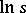,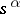are chosen for whichis real andfor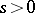.

Stable distributions, like infinitely-divisible distributions, correspond to stationary stochastic processes with stationary independent increments. A stochastically-continuous stationary stochastic process with independent incrementsis called stable if the incrementhas a stable distribution.

How to Cite This Entry:
Stable distribution. Encyclopedia of Mathematics. URL: http://encyclopediaofmath.org/index.php?title=Stable_distribution&oldid=17558
This article was adapted from an original article by B.A. Rogozin (originator), which appeared in Encyclopedia of Mathematics - ISBN 1402006098. See original article